# Capacitors in series

## Capacitors in series

Like other electrical elements, capacitors serve no purpose when used alone in a circuit. They are connected to other elements in a circuit in one of two ways: either in series or in parallel. In some cases it is useful to connect several capacitors in series in order to make a functional block:## Analysis

When this block is connected to a voltage source, each capacitor in the block stores an equal amount of charge, which means that the total amount of charge is evenly distributed across all of the capacitors, regardless of their capacitance. The amount of charge stored at each capacitor equals: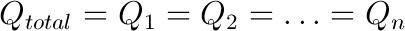where Qtotal is the total amount of charge in the complete block, and Q1 to Qn are charges at each individual capacitor.

In order to explain why the charges at every capacitor are mutually equal, and equal to the total amount of charge stored in the complete series connection block, let us assume that all capacitors were uncharged at one point in time. When voltage is first applied across the block, the same current flows through all the capacitors and as a result, charge shift occurs. Electrons are carried from one plate of each capacitor to the other, which means that the charge stored by a plate of any of the capacitors must have come from the adjacent capacitor’s plate. This means that charge carriers (electrons) have simply shifted through all the capacitors, which is the reason that the charges at each capacitor are equal.

That being said, it must be noted that the voltages across each capacitor are not equal, and are calculated for each capacitor by using the known formula: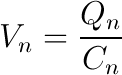where Qn is the amount of charge on every capacitor in the series connection, Cn is the capacitance of the capacitor, and Vn is the voltage across the capacitor.

By applying the Kirchoff’s Voltage Law to the series connection block, the voltage across the block equals the sum of the voltages across individual capacitors: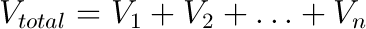By substituting the above formula for the voltage across a capacitor into this equation, having in mind that the charge at every capacitor equals the total charge stored in the series connection, we obtain the following formula: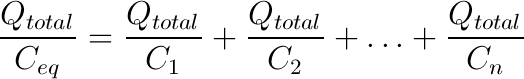which gives: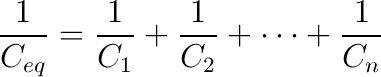where Ceq is the equivalent capacitance, and C1 to Cn are the capacitance values of every individual capacitor in the series connection.

In other words, the reciprocal value of the equivalent capacitance is equal to the sum of reciprocal capacitance values for each capacitor in the series connection.

## Applications

### Capacitive voltage divider

A voltage divider is a device which divides the applied voltage into two or more voltage outputs at a given ratio. They can be constructed using resistors or reactive elements such as capacitors. When capacitors are connected in series and a voltage is applied across this connection, the voltages across each capacitor are generally not equal, but depend on the capacitance values.

More precisely, the ratio of the voltages across individual capacitors is the inverse of the ratio of the capacitance values of each individual capacitor in the series. It should be noted that for practical purposes, capacitive voltage dividers are only useful in AC circuits, since capacitors do not pass DC signals, and also because they have a certain voltage leakage. The following figure illustrates the capacitive voltage divider: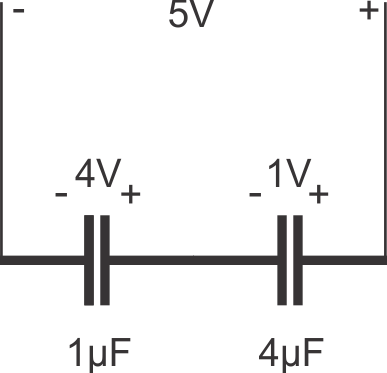### Higher working voltage

It is sometimes desirable to use a series connection of capacitors in order to be able to work with higher voltages. For example, let us assume that a 5kV power supply needs to be filtered using capacitors, and that the only available capacitors are rated at 1kV and are all of identical capacitance values. In this case, by connecting five or more such capacitors in series, the high voltage would be divided across all the capacitors and the maximum rating would not be exceeded.

Another example for the use of serially connected capacitors is a possible replacement of a car battery with a capacitor bank made of supercapacitors. Since their maximum rated voltage is only about 2.7 volts, by connecting six such supercapacitors in series, the operating voltage of the bank is raised to levels acceptable for automotive use. The advantage to using supercapacitors over a battery is the weight benefit, however this technology still needs improvement due to leakage currents and much higher capacities offered by batteries.

## Safety

A certain safety margin should be introduced when using the series capacitor circuit, and more than five capacitors would be used in a good design for this example due to two reasons. The first reason is that capacitors have a certain capacitance tolerance, and they cannot be made to identical capacitance values. As a consequence, a capacitor with a slightly smaller capacitance value would be exposed to a slightly larger voltage than the maximum rate voltage in this example. The second reason is that in reality capacitors sometimes fail. If one of the capacitors failed and became a short-circuit, the applied voltage of 5kV would be divided across the remaining 4 capacitors rated at only 1kV, and this would cause a cascade of failures, eventually shorting the voltage source and resulting in the complete destruction of the filter.